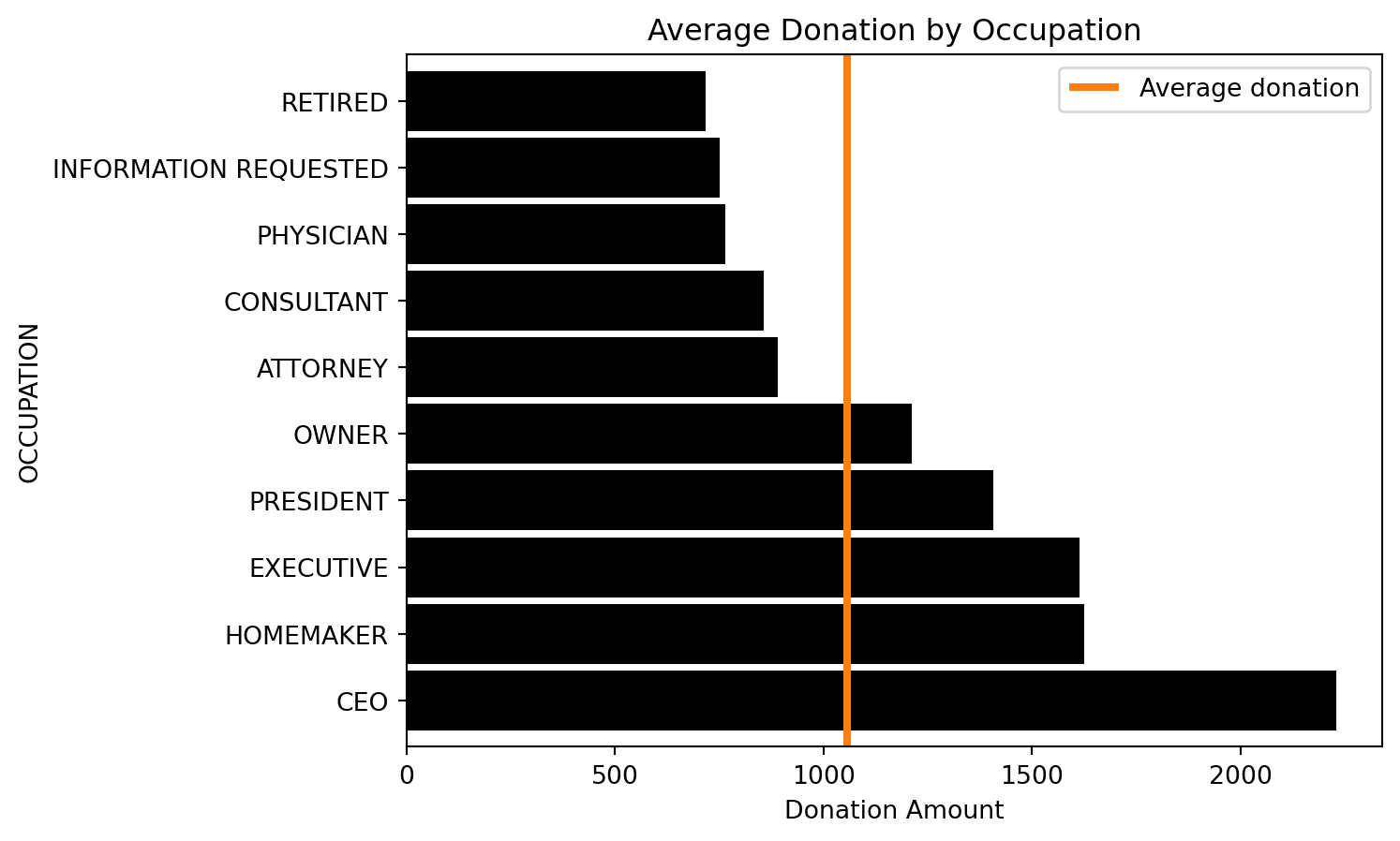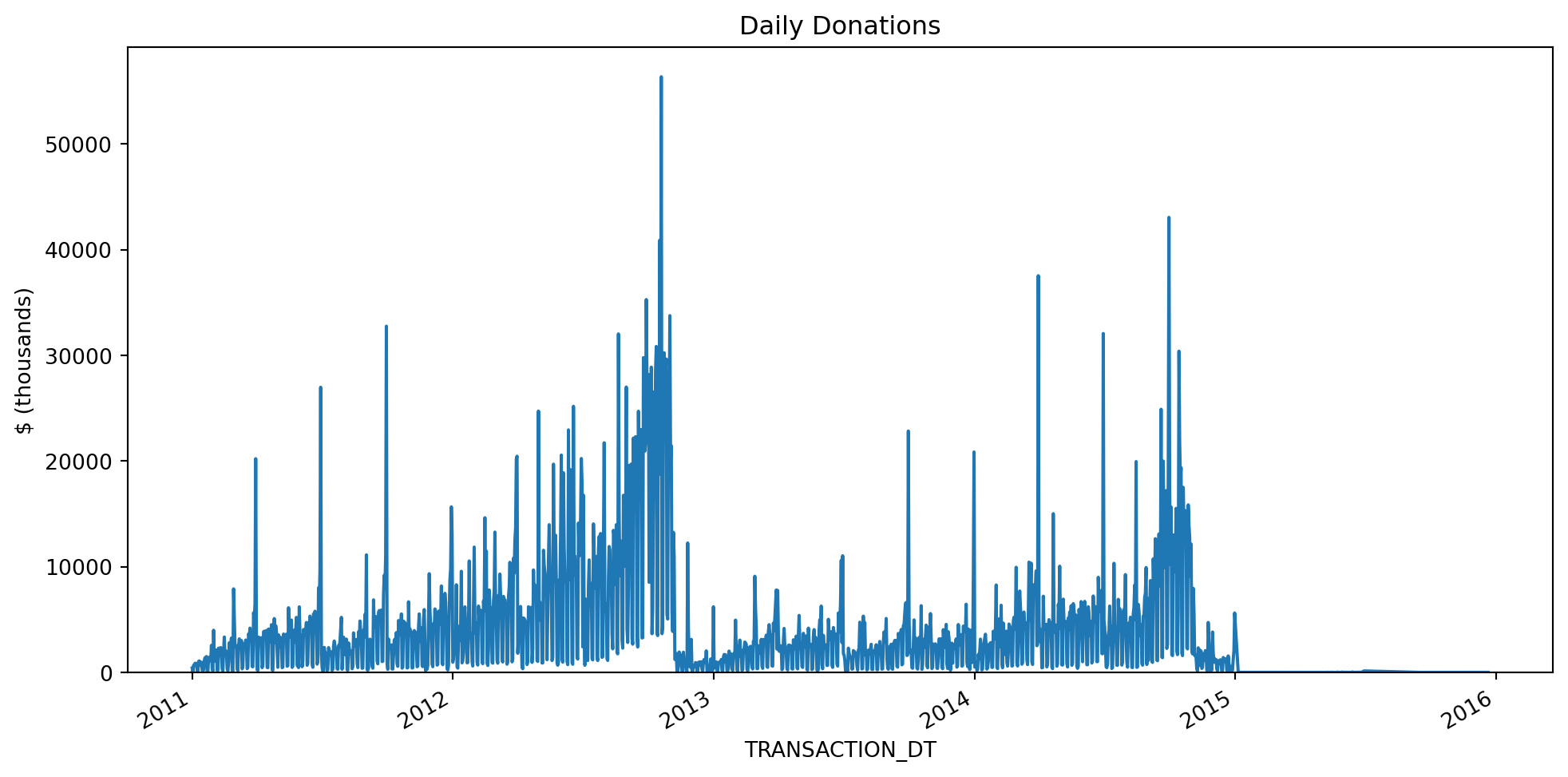In this chapter we’ll mostly compare Polars to Dask rather than to Pandas. This isn’t an apples-to-apples comparison, because Dask helps scale Pandas but it might help scale Polars too one day. Dask, like Spark, can run on a single node or on a cluster with thousands of nodes.

Polars doesn’t come with any tooling for running on a cluster, but it does have a streaming mode for larger-than-memory datasets on a single machine. It also uses memory more efficiently than Pandas. These two things mean you can use Polars for much bigger data than Pandas can handle, and hopefully you won’t need tools like Dask or Spark until you’re actually running on a cluster.

Note

I use “Dask” here as a shorthand for `dask.dataframe`. Dask does a bunch of other stuff too.

Warning

The streaming features of Polars are very new at the time of writing, so approach with caution!

## 6.1 Get the data

We’ll be using political donation data from the FEC. Warning: this takes a few minutes.

``````import asyncio
from zipfile import ZipFile
from pathlib import Path
from datetime import date
from io import BytesIO
import httpx
import polars as pl
import pandas as pd

pl.Config.set_tbl_rows(5)
pd.options.display.max_rows = 5

fec_dir = Path("../data/fec")

cm_cols = ["CMTE_ID", "CMTE_NM", "CMTE_PTY_AFFILIATION"]
dtypes = {"CMTE_PTY_AFFILIATION": pl.Categorical}
resp = await client.get(url)
with ZipFile(BytesIO(resp.content)) as z:
columns=[0, 1, 10],
new_columns=cm_cols,
sep="|",
dtypes=dtypes,
).write_parquet(fec_dir / f"cm{year}.pq")

dtypes = {
"CMTE_ID": pl.Utf8,
"EMPLOYER": pl.Categorical,
"OCCUPATION": pl.Categorical,
"TRANSACTION_DT": pl.Utf8,
"TRANSACTION_AMT": pl.Int32,
}
resp = await client.get(url)
with ZipFile(BytesIO(resp.content)) as z:
columns=[0, 11, 12, 13, 14],
new_columns=list(dtypes.keys()),
sep="|",
dtypes=dtypes,
encoding="cp1252",
).with_columns(
pl.col("TRANSACTION_DT").str.strptime(pl.Date, fmt="%m%d%Y", strict=False)
).write_parquet(
fec_dir / f"indiv{year}.pq"
)

years = ["08", "10", "12", "14", "16"]
if not fec_dir.exists():
fec_dir.mkdir()
async with httpx.AsyncClient(follow_redirects=True, timeout=None) as client:

## 6.2 Simple aggregation

Suppose we want to find the most common occupations among political donors. Let’s assume that this data is too big for your machine’s memory to read it in all at once.

We can solve this using Polars streaming, using Dask’s lazy dataframe or simply using Pandas to read the files one by one and keeping a running total:

``````# otherwise we can't read categoricals from multiple files
pl.enable_string_cache()
occupation_counts_pl = (
pl.scan_parquet(fec_dir / "indiv*.pq", cache=False)
.select(pl.col("OCCUPATION").value_counts(parallel=True, sort=True))
.collect(streaming=True)
)
occupation_counts_pl``````
shape: (579_159, 1)
OCCUPATION
struct
{"RETIRED",4773715}
{"NOT EMPLOYED",2715939}
{"PROFESSOR OF PYSICS",1}
{"ARTIST/SINGER-SONGWRITER",1}
``````import dask.dataframe as dd
fec_dir / "indiv*.pq", engine="pyarrow", columns=["OCCUPATION"]
)["OCCUPATION"].value_counts()
occupation_counts_dd.compute()``````
``````OCCUPATION
RETIRED                               4773715
NOT EMPLOYED                          2715939
...
TRANSPORTATION COMPLIANCE DIRECTOR          1
PROASSURANCE                                1
Name: count, Length: 579158, dtype: int64``````
``````files = sorted(fec_dir.glob("indiv*.pq"))

total_counts_pd = pd.Series(dtype="int64")

for year in files:
counts = occ_pd["OCCUPATION"].value_counts()

total_counts_pd.nlargest(100)``````
``````OCCUPATION
RETIRED         4773715
NOT EMPLOYED    2715939
...
SURGEON           25545
OPERATOR          25161
Length: 100, dtype: int64``````
Note

Polars can handle some larger-than-memory data even without streaming. Thanks to predicate pushdown, we can filter dataframes without reading all the data into memory first. So `streaming` mode is most useful for cases where we really do need to read in a lot of data.

## 6.3 Executing multiple queries in parallel

Often we want to generate multiple insights from the same data, and we need them in separate dataframes. In this case, using `collect_all` is more efficient than calling `.collect` multiple times, because Polars can avoid repeating common operations like reading the data.

Let’s compute the average donation size, the total donated by employer and the average donation by occupation:

``````%%time
indiv_pl = pl.scan_parquet(fec_dir / "indiv*.pq")
avg_transaction_lazy_pl = indiv_pl.select(pl.col("TRANSACTION_AMT").mean())
total_by_employer_lazy_pl = (
indiv_pl.drop_nulls("EMPLOYER")
.group_by("EMPLOYER")
.agg([pl.col("TRANSACTION_AMT").sum()])
.sort("TRANSACTION_AMT", descending=True)
)
avg_by_occupation_lazy_pl = (
indiv_pl.group_by("OCCUPATION")
.agg([pl.col("TRANSACTION_AMT").mean()])
.sort("TRANSACTION_AMT", descending=True)
)

avg_transaction_pl, total_by_employer_pl, avg_by_occupation_pl = pl.collect_all(
[avg_transaction_lazy_pl, total_by_employer_lazy_pl, avg_by_occupation_lazy_pl],
streaming=True,
comm_subplan_elim=False, # cannot use CSE with streaming
)``````
``````CPU times: user 11.8 s, sys: 2.37 s, total: 14.2 s
Wall time: 4.77 s``````
``````%%time
indiv_dd = (
# pandas and dask want datetimes but this is a date col
.assign(
TRANSACTION_DT=lambda df: dd.to_datetime(df["TRANSACTION_DT"], errors="coerce")
)
)
avg_transaction_lazy_dd = indiv_dd["TRANSACTION_AMT"].mean()
total_by_employer_lazy_dd = (
indiv_dd.groupby("EMPLOYER", observed=True)["TRANSACTION_AMT"].sum().nlargest(10)
)
avg_by_occupation_lazy_dd = (
indiv_dd.groupby("OCCUPATION", observed=True)["TRANSACTION_AMT"].mean().nlargest(10)
)
avg_transaction_dd, total_by_employer_dd, avg_by_occupation_dd = compute(
avg_transaction_lazy_dd, total_by_employer_lazy_dd, avg_by_occupation_lazy_dd
)``````
``````CPU times: user 22.4 s, sys: 3.28 s, total: 25.7 s
Wall time: 15.3 s``````

The Polars code above tends to be ~3.5x faster than Dask on my machine, which if anything is a smaller speedup than I expected.

We should also profile memory usage, since it could be the case that Polars is just running faster because it’s reading in bigger chunks. According to the `fil` profiler, the Dask example’s memory usage peaks at 1450 MiB, while Polars uses ~10% more than that.

Before I forget, here are the results of our computations:

### 6.3.1 avg_transaction

``avg_transaction_pl``
shape: (1, 1)
TRANSACTION_AMT
f64
563.97184
``avg_transaction_dd``
``563.9718398183915``

### 6.3.2 total_by_employer

``total_by_employer_pl``
shape: (10, 2)
EMPLOYERTRANSACTION_AMT
cati32
"RETIRED"1023306104
"SELF-EMPLOYED"834757599
"FAHR, LLC"166679844
"CANDIDATE"75187243
``total_by_employer_dd``
``````EMPLOYER
RETIRED          1023306104
SELF-EMPLOYED     834757599
...
FAHR, LLC         166679844
CANDIDATE          75187243
Name: TRANSACTION_AMT, Length: 10, dtype: int32``````

### 6.3.3 avg_by_occupation

``avg_by_occupation_pl``
shape: (10, 2)
OCCUPATIONTRANSACTION_AMT
catf64
"CHAIRMAN CEO &…1.0233e6
"PAULSON AND CO…1e6
"PERRY HOMES"500000.0
"CHIEF EXECUTIV…500000.0
``avg_by_occupation_dd``
``````OCCUPATION
CHAIRMAN CEO & FOUNDER              1.023333e+06
PAULSON AND CO., INC.               1.000000e+06
...
OWNER, FOUNDER AND CEO              5.000000e+05
CHIEF EXECUTIVE OFFICER/PRODUCER    5.000000e+05
Name: TRANSACTION_AMT, Length: 10, dtype: float64``````

## 6.4 Filtering

Let’s filter for only the 10 most common occupations and compute some summary statistics:

### 6.4.1 avg_by_occupation, filtered

Getting the most common occupations:

``````top_occupations_pl = (
occupation_counts_pl.select(
pl.col("OCCUPATION")
.struct.field("OCCUPATION")
.drop_nulls()
)
.to_series()
)
top_occupations_pl``````
shape: (10,)
OCCUPATION
cat
"RETIRED"
"NOT EMPLOYED"
"EXECUTIVE"
"ENGINEER"
``````top_occupations_dd = occupation_counts_dd.head(10).index
top_occupations_dd``````
``````CategoricalIndex(['RETIRED', 'NOT EMPLOYED', 'ATTORNEY', 'PHYSICIAN',
'HOMEMAKER', 'PRESIDENT', 'PROFESSOR', 'CONSULTANT',
'EXECUTIVE', 'ENGINEER'],
categories=['PUBLIC RELATIONS CONSULTANT', 'PRESIDENT', 'PHYSICIAN', 'SENIOR EXECUTIVE', ..., 'VICE PRESIDENT - FUEL PROCUREMENT', 'INFORMATION TECHNOLOGY SPECI', 'SR MANAGER, PROJECT PLANNING', 'PROASSURANCE'], ordered=False, dtype='category', name='OCCUPATION')``````
``````donations_pl_lazy = (
indiv_pl.filter(pl.col("OCCUPATION").is_in(top_occupations_pl.to_list()))
.group_by("OCCUPATION")
.agg(pl.col("TRANSACTION_AMT").mean())
)
total_avg_pl, occupation_avg_pl = pl.collect_all(
[indiv_pl.select(pl.col("TRANSACTION_AMT").mean()), donations_pl_lazy],
streaming=True,
comm_subplan_elim=False
)``````
``````donations_dd_lazy = (
indiv_dd[indiv_dd["OCCUPATION"].isin(top_occupations_dd)]
.groupby("OCCUPATION", observed=True)["TRANSACTION_AMT"]
.mean()
.dropna()
)
total_avg_dd, occupation_avg_dd = compute(
indiv_dd["TRANSACTION_AMT"].mean(), donations_dd_lazy
)``````

### 6.4.2 Plotting

These results are small enough to plot:

``````ax = (
occupation_avg_pl
.to_pandas()
.set_index("OCCUPATION")
.squeeze()
.sort_values(ascending=False)
.plot.barh(color="k", width=0.9)
)
lim = ax.get_ylim()
ax.vlines(total_avg_pl, *lim, color="C1", linewidth=3)
ax.legend(["Average donation"])
ax.set(xlabel="Donation Amount", title="Average Donation by Occupation")``````
``````[Text(0.5, 0, 'Donation Amount'),
Text(0.5, 1.0, 'Average Donation by Occupation')]````````````ax = occupation_avg_dd.sort_values(ascending=False).plot.barh(color="k", width=0.9)
lim = ax.get_ylim()
ax.vlines(total_avg_dd, *lim, color="C1", linewidth=3)
ax.legend(["Average donation"])
ax.set(xlabel="Donation Amount", title="Average Donation by Occupation")``````
``````[Text(0.5, 0, 'Donation Amount'),
Text(0.5, 1.0, 'Average Donation by Occupation')]``````## 6.5 Resampling

Resampling is another useful way to get our data down to a manageable size:

``````daily_pl = (
indiv_pl.select(["TRANSACTION_DT", "TRANSACTION_AMT"])
.drop_nulls()
.sort("TRANSACTION_DT")
.group_by_dynamic("TRANSACTION_DT", every="1d")
.agg(pl.col("TRANSACTION_AMT").sum())
.filter(
pl.col("TRANSACTION_DT")
.is_between(date(2011, 1, 1), date(2017, 1, 1), closed="left")
)
.with_columns(pl.col("TRANSACTION_AMT") / 1000)
.collect(streaming=True)
)
ax = (
daily_pl.select(
[pl.col("TRANSACTION_DT").cast(pl.Datetime), "TRANSACTION_AMT"]
)
.to_pandas()
.set_index("TRANSACTION_DT")
.squeeze()
.plot(figsize=(12, 6))
)
ax.set(ylim=0, title="Daily Donations", ylabel="\$ (thousands)")``````
``````[(0.0, 83407.5242),
Text(0.5, 1.0, 'Daily Donations'),
Text(0, 0.5, '\$ (thousands)')]````````````daily_dd = (
indiv_dd[["TRANSACTION_DT", "TRANSACTION_AMT"]]
.dropna()
.set_index("TRANSACTION_DT")["TRANSACTION_AMT"]
.resample("D")
.sum()
.loc["2011":"2016"]
.div(1000)
.compute()
)

ax = daily_dd.plot(figsize=(12, 6))
ax.set(ylim=0, title="Daily Donations", ylabel="\$ (thousands)")``````
``````/home/user/mambaforge/envs/modern-polars/lib/python3.11/site-packages/partd/pandas.py:138: FutureWarning: is_datetime64tz_dtype is deprecated and will be removed in a future version. Check `isinstance(dtype, pd.DatetimeTZDtype)` instead.
elif is_datetime64tz_dtype(block):``````
``````/home/user/mambaforge/envs/modern-polars/lib/python3.11/site-packages/partd/pandas.py:138: FutureWarning: is_datetime64tz_dtype is deprecated and will be removed in a future version. Check `isinstance(dtype, pd.DatetimeTZDtype)` instead.
elif is_datetime64tz_dtype(block):``````
``````[(0.0, 83407.5242),
Text(0.5, 1.0, 'Daily Donations'),
Text(0, 0.5, '\$ (thousands)')]``````## 6.6 Joining

Polars joins work in streaming mode. Let’s add join the donations data with the committee master data, which contains information about the committees people donate to.

``````cm_pl = (
# This data is small so we don't use streaming.
# Also, .last isn't available in lazy mode.
# Some committees change their name, but the ID stays the same
.group_by("CMTE_ID", maintain_order=True).last()
)
cm_pl``````
shape: (28_467, 3)
CMTE_IDCMTE_NMCMTE_PTY_AFFILIATION
strstrcat
"C00000042""ILLINOIS TOOL …null
"C00000059""HALLMARK CARDS…"UNK"
"C90017336""LUDWIG, EUGENE…null
"C90017542""CENTER FOR POP…null
``````cm_dd = (
# This data is small but we use dask here as a
# convenient way to read a glob of files.
.compute()
# Some committees change their name, but the
# ID stays the same.
# If we use .last instead of .nth(-1),
# we get the last non-null value
.groupby("CMTE_ID", as_index=False)
.nth(-1)
)
cm_dd``````
CMTE_ID CMTE_NM CMTE_PTY_AFFILIATION
7 C00000794 LENT & SCRIVNER PAC UNK
15 C00001156 MICHIGAN LEAGUE OF COMMUNITY BANKS POLITICAL A... NaN
... ... ... ...
17649 C99002396 AMERICAN POLITICAL ACTION COMMITTEE NaN
17650 C99003428 THIRD DISTRICT REPUBLICAN PARTY REP

28467 rows × 3 columns

Merging:

``````indiv_filtered_pl = indiv_pl.filter(
pl.col("TRANSACTION_DT").is_between(
date(2007, 1, 1), date(2017, 1, 1), closed="both"
)
)
merged_pl = indiv_filtered_pl.join(cm_pl.lazy(), on="CMTE_ID")``````
``````indiv_filtered_dd = indiv_dd[
(indiv_dd["TRANSACTION_DT"] >= pd.Timestamp("2007-01-01"))
& (indiv_dd["TRANSACTION_DT"] <= pd.Timestamp("2017-01-01"))
]
merged_dd = dd.merge(indiv_filtered_dd, cm_dd, on="CMTE_ID")``````

Daily donations by party:

``````party_donations_pl = (
merged_pl.group_by(["TRANSACTION_DT", "CMTE_PTY_AFFILIATION"])
.agg(pl.col("TRANSACTION_AMT").sum())
.sort(["TRANSACTION_DT", "CMTE_PTY_AFFILIATION"])
.collect(streaming=True)
)``````
``````party_donations_dd = (
(
merged_dd.groupby(["TRANSACTION_DT", "CMTE_PTY_AFFILIATION"])[
"TRANSACTION_AMT"
].sum()
)
.compute()
.sort_index()
)``````

Plotting daily donations:

``````ax = (
party_donations_pl
.pivot(
index="TRANSACTION_DT", columns="CMTE_PTY_AFFILIATION", values="TRANSACTION_AMT"
)[1:, :]
.select(
[pl.col("TRANSACTION_DT"), pl.col(pl.Int32).rolling_mean(30, min_periods=0)]
)
.to_pandas()
.set_index("TRANSACTION_DT")
[["DEM", "REP"]]
.plot(color=["C0", "C3"], figsize=(12, 6), linewidth=3)
)
ax.set(title="Daily Donations (30-D Moving Average)", xlabel="Date")``````
``[Text(0.5, 1.0, 'Daily Donations (30-D Moving Average)'), Text(0.5, 0, 'Date')]````````ax = (
party_donations_dd
.unstack("CMTE_PTY_AFFILIATION")
.iloc[1:]
.rolling("30D")
.mean()
[["DEM", "REP"]]
.plot(color=["C0", "C3"], figsize=(12, 6), linewidth=3)
)
ax.set(title="Daily Donations (30-D Moving Average)", xlabel="Date")``````
``[Text(0.5, 1.0, 'Daily Donations (30-D Moving Average)'), Text(0.5, 0, 'Date')]``